# CPU Scheduling | Operating System

In this article, we will learn about CPU Scheduling criteria, and CPU Scheduling algorithms in Operating System.
Submitted by Abhishek Kataria, on July 24, 2018

## Scheduling Criteria

There are many criteria which have been suggested for comparing the CPU scheduling algorithms. The characteristics which are used for comparison and then used to determine the best algorithms, for this some of the criteria are given below:

1. CPU utilization
This is the main task in which we have to keep the CPU as busy as possible. Its known, that CPU utilization may range from 0 to 100 percent, but in a case of the real system it should range from 40 percent for the low-level system to 90 percent for high level used system.
2. Throughput
The number of processes which complete their execution per unit time it is called Throughput. In the case of CPU scheduling, CPU is busy in executing the process, then the work is being done, and the work completed per unit time can also be called as throughput.
3. Turnaround Time
This is an amount of time to execute a particular process. Turnaround time is the sum of the periods spent waiting to get into memory, executing on the CPU, waiting in the queue and doing I/O i.e. the interval between the time of submission of a process to the time of completion is the turnaround time.
4. Waiting time
It is an amount of time in which a process has been waiting in the ready queue. It only affects the amount of time that a process spends waiting in the ready queue.
5. Response time
It is an amount of time in which the request was submitted until the first response is produced, not output.

In all these cases we need to maximize the CPU utilization and throughput, and to minimize the turnaround time, waiting time, and respond time.

## Scheduling Algorithms

Scheduling algorithms deal with the problems of deciding the process which is in the ready queue and need to be allocated in the CPU. There are some algorithms which are discussed below:

1. First-Come First-Served Scheduling

It is a simplest CPU scheduling algorithm. According to this, the process that requests the CPU first is allocated at the CPU first. FCFS is managed with the help of the FIFO queue. When a process enters into a ready queue it will link up at the tail of the queue and when the CPU is free it allocates the process at the head of the queue and by this running, the process is removed from the queue. This is a very simple algorithm for write and to understand.

Consider the following process that arrives at a time 0 with the CPU burst time in milliseconds.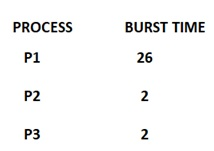If the process arrive in the order of P1, P2, P3 in order of FCFS then the Gantt chart for this will be: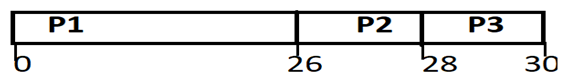i.e. average waiting time will be : (0+26+28)/3 = 18. The FCFS scheduling algorithm is non pre-emptive.

2. Shortest Job first scheduling

This is a different approach in need of CPU scheduling. According to this algorithm when the CPU is free, the process will be assigned which will have the smallest next CPU burst. SJF is optimal which means it will provide the average waiting time for a given set of processes. Let us consider an example with the following set of processes, with the length of CPU burst time given in milliseconds.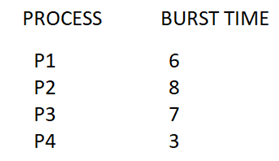Gantt chart for this will be: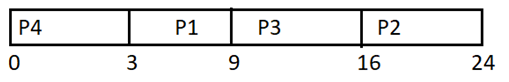i.e. average time by using SJF is (0+3+9+16)/4 =7 milliseconds. As SJF is an optimal algorithm which cannot be implemented at the level of short term CPU scheduling as there is no way to know that the length of the next CPU burst.

3. Priority Scheduling

In this kind of algorithms, a priority is associated with a process and by that CPU will be allocated to the process which will have the highest priority. By this, we can conclude that larger the CPU burst, the lower the priority and vice versa. Let us consider an example with the following set of the process with the length of the CPU burst time given in milliseconds.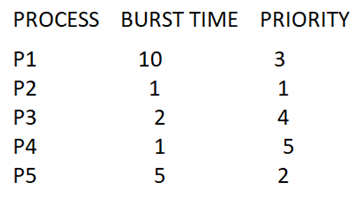In this case, the average waiting time is (0+1+6+16+18)/5 =8.2 milliseconds. Priority scheduling can be either pre-emptive or non-pre-emptive. A major problem with the priority scheduling is starvation which means low priority process never executes and the solution to the problem is aging. Aging is a technique of increasing the priority of the process which had wait in the system for a long time.

4. Round Robin Scheduling

The round robin scheduling algorithm is designed for a time-sharing system in which a small time is defined termed as time quantum. A time quantum is generally from the 10 to 100 milliseconds. For implementing the RR scheduling the CPU scheduler keeps the process for the ready queue and sets a timer to interrupt after the one-time quantum and then the process will be dispatched. If there are m processes in the queue and the time quantum is q then each process gets 1/m of the CPU time and by this, no process will wait for more than (m-1)q time units.